Next: Measures of Amplitude Up: book Previous: Preface   Contents   Index

# Sinusoids, amplitude and frequency

Electronic music is usually made using a computer, by synthesizing or processing digital audio signals. These are sequences of numbers,where the index, called the sample number, may range over some or all the integers. A single number in the sequence is called a sample. An example of a digital audio signal is the Sinusoid: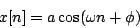where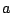is the amplitude,is the angular frequency, andis the initial phase. The phase is a function of the sample number, equal to. The initial phase is the phase at the zeroth sample (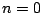).

Figure 1.1 (part a) shows a sinusoid graphically. The horizontal axis shows successive values ofand the vertical axis shows the corresponding values of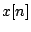. The graph is drawn in such a way as to emphasize the sampled nature of the signal. Alternatively, we could draw it more simply as a continuous curve (part b). The upper drawing is the most faithful representation of the (digital audio) sinusoid, whereas the lower one can be considered an idealization of it.Sinusoids play a key role in audio processing because, if you shift one of them left or right by any number of samples, you get another one. This makes it easy to calculate the effect of all sorts of operations on sinusoids. Our ears use this same special property to help us parse incoming sounds, which is why sinusoids, and combinations of sinusoids, can be used to achieve many musical effects.

Digital audio signals do not have any intrinsic relationship with time, but to listen to them we must choose a sample rate, usually given the variable name, which is the number of samples that fit into a second. The time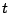is related to the sample numberby, or. A sinusoidal signal with angular frequencyhas a real-time frequency equal toin Hertz (i.e., cycles per second), because a cycle is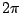radians and a second issamples.

A real-world audio signal's amplitude might be expressed as a time-varying voltage or air pressure, but the samples of a digital audio signal are unitless numbers. We'll casually assume here that there is ample numerical accuracy so that we can ignore round-off errors, and that the numerical format is unlimited in range, so that samples may take any value we wish. However, most digital audio hardware works only over a fixed range of input and output values, most often between -1 and 1. Modern digital audio processing software usually uses a floating-point representation for signals. This allows us to use whatever units are most convenient for any given task, as long as the final audio output is within the hardware's range [Mat69, pp. 4-10].

SubsectionsNext: Measures of Amplitude Up: book Previous: Preface   Contents   Index
Miller Puckette 2006-12-30